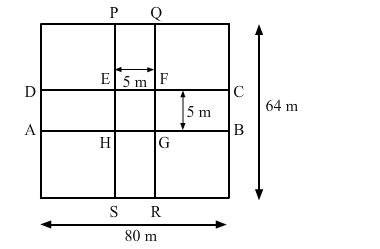# A 80 m by 64 m rectangular lawn has two roads, each 5 m wide, running through its`
Question:

A 80 m by 64 m rectangular lawn has two roads, each 5 m wide, running through its   middle, one parallel to its length and the other parallel to its breadth. Find the cost of gravelling the roads at Rs 40 per m2.

Solution:

The length and breadth of the lawn are 80 m and 64 m, respectively.
The layout of the roads is shown in the figure below:Area of the road $A B C D=80 \times 5=400 \mathrm{~m}^{2}$

Area of the road $P Q R S=64 \times 5=320 \mathrm{~m}^{2}$

Clearly, the area EFGH is common in both the roads.

Area EFGH $=5 \times 5=25 \mathrm{~m}^{2}$

Area of the roads $=400+320-25$

$=695 \mathrm{~m}^{2}$

Given:
Cost of gravelling 1 m2 area = Rs 40

$\therefore$ Cost of gravelling $695 \mathrm{~m}^{2}$ area $=695 \times 40$

= Rs 27,800